Latest Banking jobs   »   Profit and Loss

# Profit and Loss: Formulas, Concepts, Tricks, Questions & Example

Profit and Loss: Profit and loss are the terms used to find whether any transaction is profitable or not. The concept of profit & loss is used by us in our daily lives as well. Mostly we use this concept to calculate the loss or gain on any product. The price at which we buy anything is called cost price and the price at which any product is sold is called selling price. There is also a marked price which means the price which is printed on any product. So in this article, we are going to discuss profit and loss formulas, concepts, tricks, questions, and examples.

## Profit & Loss: Bank Exams

Profit & Loss is one of the favorite topics of the examiner which is commonly asked in the banking examinations. Profit & Loss is asked in the prelims as well as the mains exam with a difference in the difficulty level. Instead of doing again & again, clear your concepts once and then practice profit & loss questions. Most of the candidates make a mistake by directly jumping on the short tricks instead of learning the core concepts therefore we will provide all the formulas of profit & loss and also try to clear your concepts. Keep reading this article to check profit & loss questions & example.

## Profit and Loss: Basic Terms

Here we have provided below some of the basic formulas of profit & loss which will help you in solving questions quickly.

### Cost Price

The price at which an article is purchased is called its cost price (C.P.)

### Selling Price

The price at which the article is sold is called its selling price (S.P.)

### Profit

When the selling price is more than the cost price then it is called profit

### Loss

When the selling price is less than the cost price then it is called loss

### Marked Price or List Price (MP or LP) and Discount

Marked price is the price that is marked on the product or that is quoted in the price list. It is the price at which the product is quoted or intended to be sold. However, the seller can decide to give discounts to the buyer and the actual selling price might be different from the marked price. Given that there is no discount, the marked price is the same as the selling price.
The amount of discount given will always be calculated on the marked price. This can be expressed as      Discount Percent= MP-SP/MP×100

## Profit Loss: Formulas

• Profit/Gain = Selling Price – Cost Price
• Loss = Cost Price – Selling Price
• Cost Price = Selling Price ( No profit No loss)
• Gain Percentage = (Gain × 100)/(C.P.)
• Loss Percentage = (Loss × 100)/(C.P.)

## Profit and Loss: Tricks

Well, tricks are nothing but a way to reduce the steps to calculate the answer, if you know the basics then only you can understand the logic behind the tricks. So we have provided below some profit & loss tricks which you can use while solving similar types of questions

1. Profit, P = SP – CP; SP>CP
2. Loss, L = CP – SP; CP>SP
3. P% = (P/CP) x 100
4. L% = (L/CP) x 100
5. SP = {(100 + P%)/100} x CP
6. SP = {(100 – L%)/100} x CP
7. CP = {100/(100 + P%)} x SP
8. CP = {100/(100 – L%)} x SP
9. Discount = MP – SP
10. SP = MP -Discount
11. When two different articles are sold at the same selling price getting a gain of x% on the first and loss of x% on the second, then the overall% loss in the transaction is given by (x/10)² %. (Note: In such questions there is always a loss.)
12.  A merchant uses faulty measures and sells his goods at gain/loss of x%. The overall % gain/loss(g) is given by (100+g)/(100+x)=(True measure)/(Faulty measure). (Note: If the merchant sells his goods at cost price, then x = 0.)
13. A merchant uses y% less weight/length and sells his goods at gain/loss of x%. The overall % gain/loss is given by [((y+x)/(100-y))×100]%

## Profit & Loss: Dishonest Dealer Concept

Dishonest Dealers and faulty weights is an important topic in profit and loss generally asked in all competitive exams. In this topic, we come across such type of problems in which shopkeeper sells the items at cost price but still, he makes a profit on those items. This can be only possible if he gives less quantity to the customer then the indicated on the product. A dishonest merchant shows he is selling the product on cost price in the welfare of customer but in that he reduced its weight.
For example, if shopkeeper sells a 1-liter packet of milk at its cost price but he gives only 800 ml milk in that packet. In this case, he is giving 800 ml milk to a customer and charging amount for 1000 ml to the customer. Hence, we can say that 800 ml is cost price and 1000 ml is selling price. So, he is getting a profit of 200 ml of milk on selling 800 ml milk.

Percentage profit = ((Selling Price – Cost Price)/(Cost Price))×100
Percentage profit = ((1000-800)/800)×100 = 25%

## Profit and Loss: Questions

Q1. What will be the single discount equivalent to two successive discounts of 25% and 24%?
(a) 46%
(b) 43%
(c) 40%
(d) 33%
(e) 49%

Q2. I bought 16 pencils at the rate of Rs 9 per dozen and sold all of them at the rate of Rs 12 per dozen. What is the overall profit percentage in this transaction?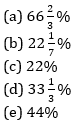Q3. A seller marks up an article 160% above of its cost price. After it he allows two successive discount of 20% and 25% and yet he gets 56% profit on it. If marked price of article is Rs 520 then find its selling price
(a) Rs 320
(b) Rs 312
(c) Rs 240
(d) Rs 324
(e) Rs 230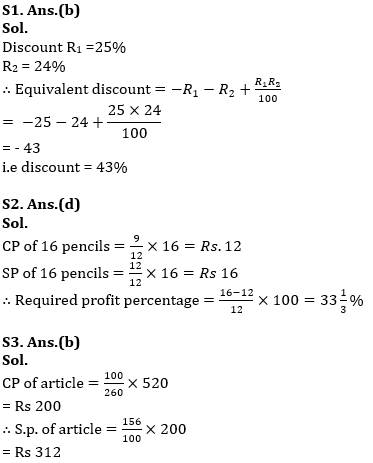Q4. A milkman buys some milk. If he sells it at Rs 5 per litre, he loses Rs 300, but when he sells it at Rs 6 per litre, he gains Rs 250. How much milk did he purchase?
(a) 550 lt
(b) 300 lt
(c) 250 lt
(d) 800 lt
(e) 650 lt

Q5. A trader sells two articles for Rs. 4,800 each, neither losing nor gaining in total. If he sold one of the articles at a gain of 20%, the other is sold at a loss of what percent?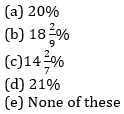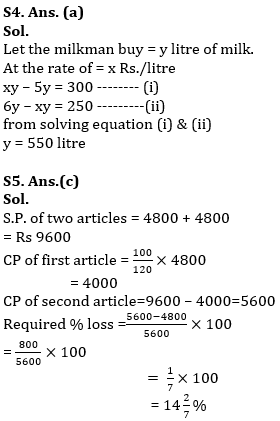Q6. How many kg of tea worth Rs. 25 per kg must be blended with 30 kg of tea worth Rs. 30 per kg so that by selling the blended variety at Rs. 30 per kg there should be gain of 10%?
(a) 30 kg
(b) 32 kg
(c) 36 kg
(d) 42 kg
(e) 34 kg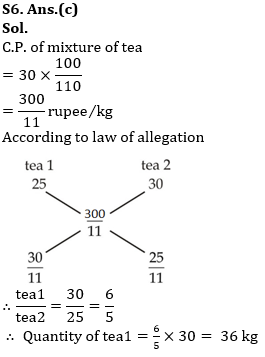Q7. The price of a product after getting 10% discount is Rs.9450 which includes 5% tax on selling price. Find the marked price of the product (in Rs)?
(a) 8500
(b) 9000
(c) 10000
(d) 9500
(e) 10500

Q8. A shopkeeper in place of selling a article at 10% profit, mark up the article 30% above cost price and gives a discount of 10%. In this process he gets Rs. 56 more profit. Find the cost price of article.
(a) Rs. 400
(b) Rs. 1000
(c) Rs. 800
(d) Rs. 600
(e) Rs. 500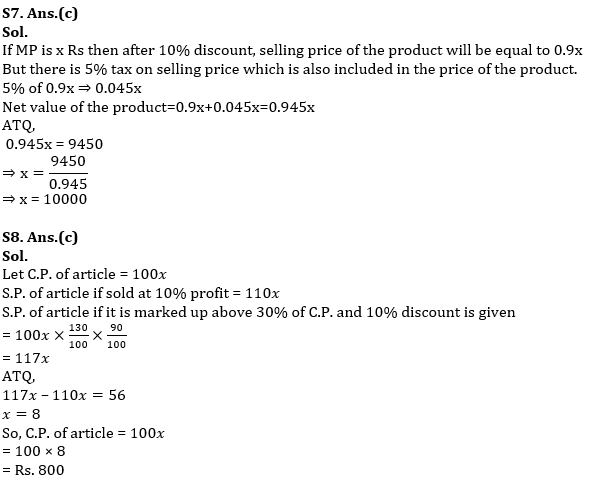Q9. A person purchases four bicycles at a discount of 20% on marked price on each bicycle. If sum of discount offered and profit obtained on all 4 bicycles is 2560 then find the difference in marked price and cost price on one bicycle.
(a) 520
(b) 640
(c) 1220
(d) 880
(e) 690

Q10. Veer buy an article for Rs. 480. He sold it at 12% loss and get some money and from that money he again buys an article and this he sold at 25% profit. What was profit percentage he got from this transaction?
(a) 16%
(b) 12%
(c) 10%
(d) 14%
(e) 20%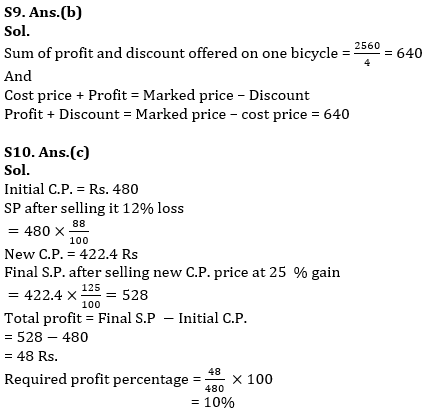Profit and Loss: Formulas, Concepts, Tricks, Questions &amp; Example in Hindi## FAQs: Profit and Loss

Q.1 What is profit & loss?
Ans Profit and Loss is used in mathematics to determine the price of a commodity in the market and understand how profitable a business is

Q.2 What is cost price and selling price in profit & loss?
Ans The price at which any product is purchased is called cost price and the price at which any product is sold is called selling price

Q.3 What is discount formula in profit & loss ?
Ans The formula to calculate to discount is Marked price – Selling price.

Q.4 What is the formula of CP and SP?
Ans The formula of CP and SP is CP = {100/(100 + P%)} x SP and SP = MP -Discount

Q.5 How do you calculate profit or loss?
Ans Profit can be calculated by deducting cost price from selling price while loss can be calculated by deducting selling price from cost price.

Q.6 What is profit & loss percentage formula?
Ans The profit & loss percentage formula is P% = (P/CP) x 100 and L% = (L/CP) x 100

#### Congratulations!Incorrect details? Fill the form again here

•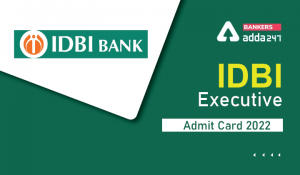IDBI Executive Admit Card 2022 Out, Call...
•IBPS RRB PO Cut Off 2022, Previous Year ...
•NABARD SO Recruitment 2022 Notification ...
•Uttarakhand GDS Result 2022 Out, UK GDS ...
•Can 12th Pass Apply For IBPS RRB?
•Quantitative Aptitude Quiz For IBPS RRB ...